## Harmonic Oscillator Solution with Operators

We can solve the harmonic oscillator problem using operator methods. We write the Hamiltonian in terms of the operator.We compute the commutators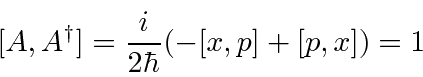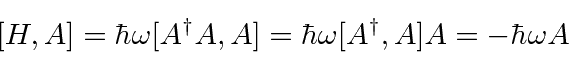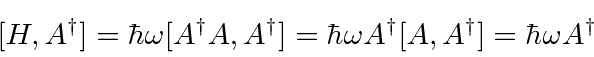If we apply the the commutator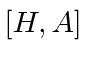to the eigenfunction, we get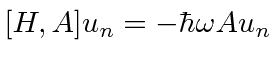which rearranges to the eigenvalue equationThis says thatis an eigenfunction of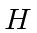with eigenvalueso it lowers the energy by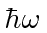. Since the energy must be positive for this Hamiltonian, the lowering must stop somewhere, at the ground state, where we will haveThis allows us to compute the ground state energy like thisshowing that the ground state energy is. Similarly,raises the energy by. We can travel up and down the energy ladder usingand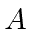, always in steps of. The energy eigenvalues are therefore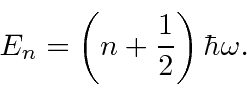A little more computation shows that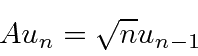and thatThese formulas are useful for all kinds of computations within the important harmonic oscillator system. Bothandcan be written in terms ofand.Jim Branson 2013-04-22